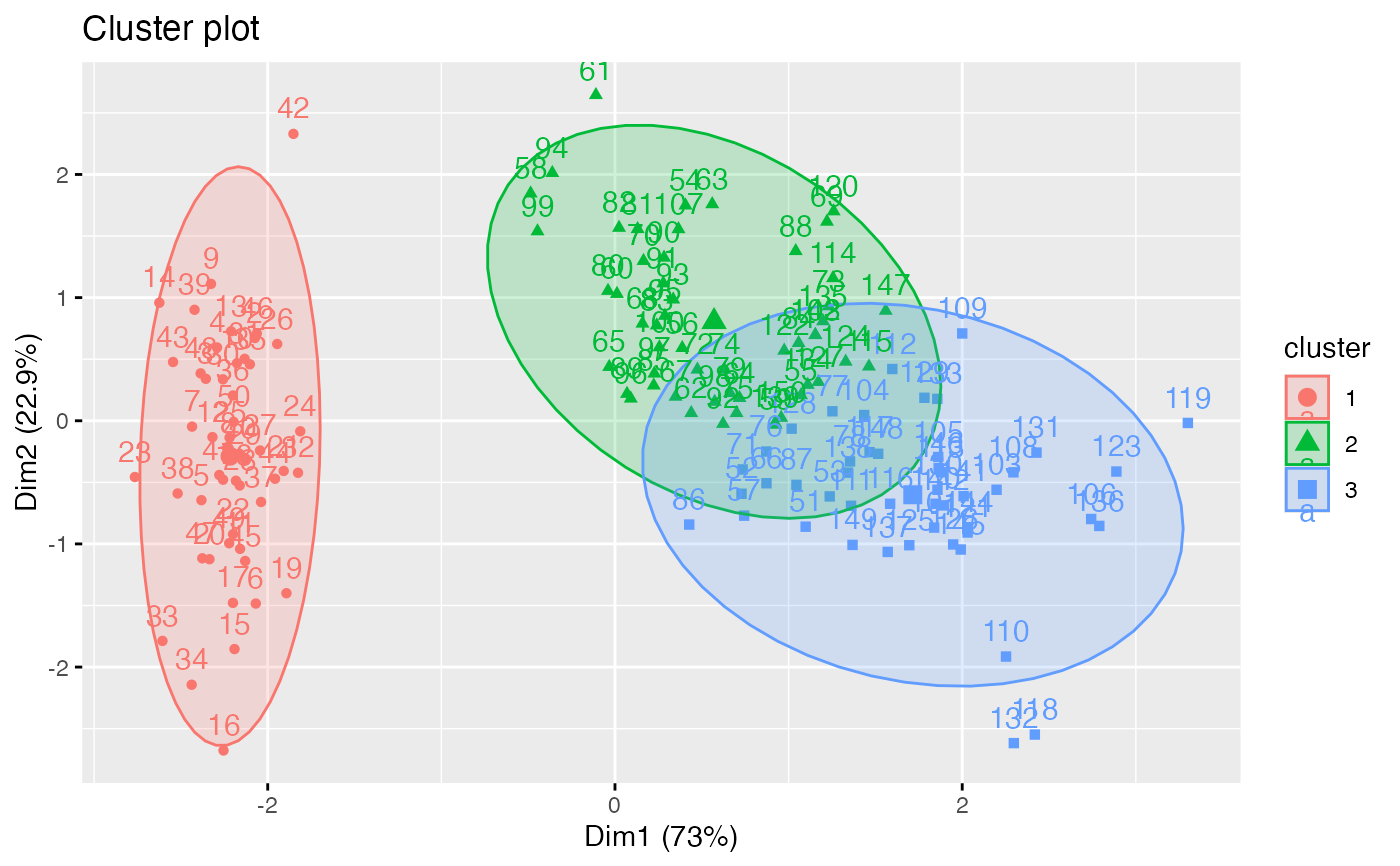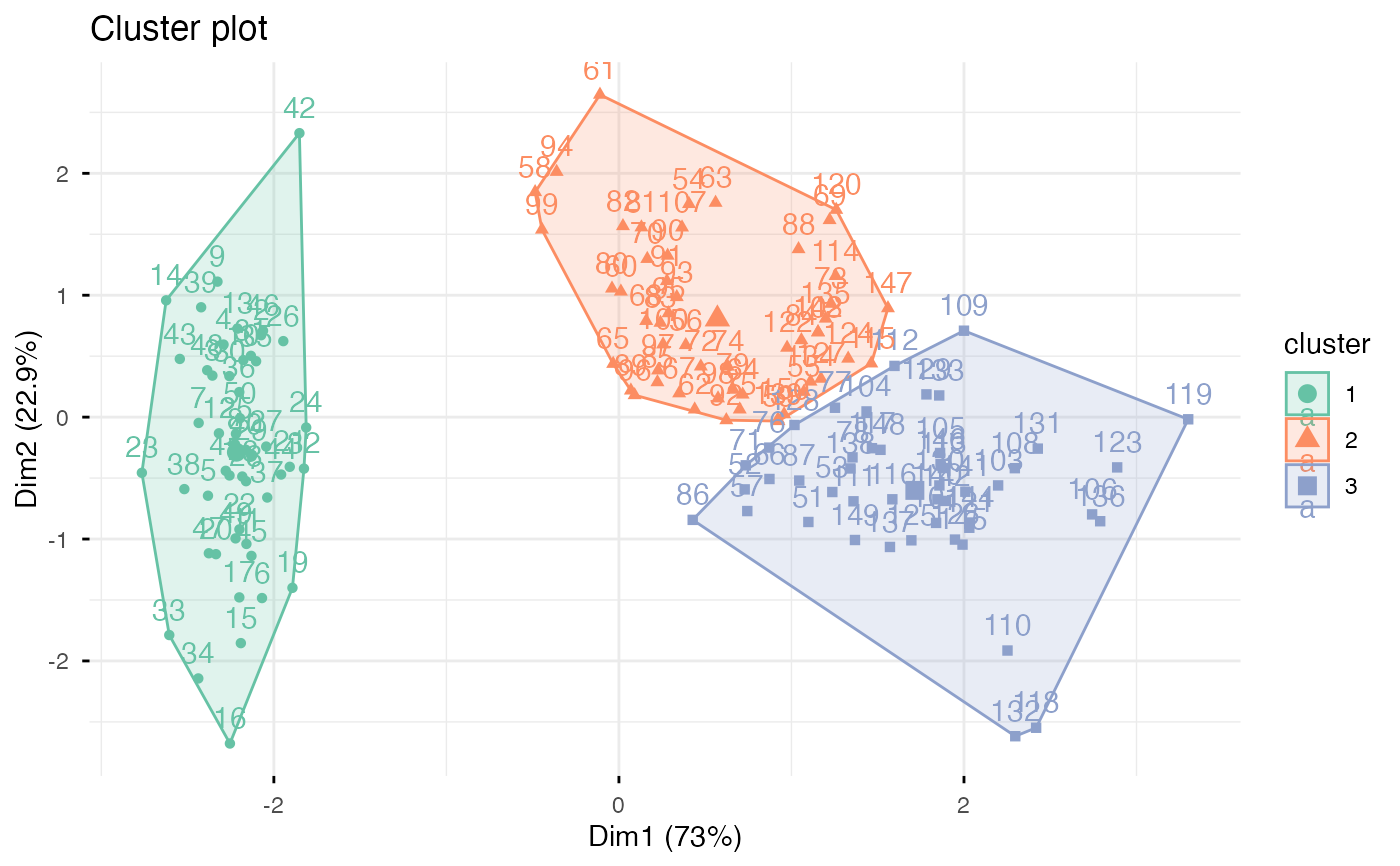Provides ggplot2-based elegant visualization of partitioning methods including kmeans [stats package]; pam, clara and fanny [cluster package]; dbscan [fpc package]; Mclust [mclust package]; HCPC [FactoMineR]; hkmeans [factoextra]. Observations are represented by points in the plot, using principal components if ncol(data) > 2. An ellipse is drawn around each cluster.

fviz_cluster(object, data = NULL, choose.vars = NULL, stand = TRUE,
axes = c(1, 2), geom = c("point", "text"), repel = FALSE,
show.clust.cent = TRUE, ellipse = TRUE, ellipse.type = "convex",
ellipse.level = 0.95, ellipse.alpha = 0.2, shape = NULL,
pointsize = 1.5, labelsize = 12, main = "Cluster plot",
xlab = NULL, ylab = NULL, outlier.color = "black",
outlier.shape = 19, outlier.pointsize = pointsize,
outlier.labelsize = labelsize, ggtheme = theme_grey(), ...)

## Arguments

object an object of class "partition" created by the functions pam(), clara() or fanny() in cluster package; "kmeans" [in stats package]; "dbscan" [in fpc package]; "Mclust" [in mclust]; "hkmeans", "eclust" [in factoextra]. Possible value are also any list object with data and cluster components (e.g.: object = list(data = mydata, cluster = myclust)). the data that has been used for clustering. Required only when object is a class of kmeans or dbscan. a character vector containing variables to be considered for plotting. logical value; if TRUE, data is standardized before principal component analysis a numeric vector of length 2 specifying the dimensions to be plotted. a text specifying the geometry to be used for the graph. Allowed values are the combination of c("point", "text"). Use "point" (to show only points); "text" to show only labels; c("point", "text") to show both types. a boolean, whether to use ggrepel to avoid overplotting text labels or not. logical; if TRUE, shows cluster centers logical value; if TRUE, draws outline around points of each cluster Character specifying frame type. Possible values are 'convex', 'confidence' or types supported by stat_ellipse including one of c("t", "norm", "euclid"). the size of the concentration ellipse in normal probability. Passed for ggplot2::stat_ellipse 's level. Ignored in 'convex'. Default value is 0.95. Alpha for frame specifying the transparency level of fill color. Use alpha = 0 for no fill color. the shape of points. the size of points font size for the labels plot main title. character vector specifying x and y axis labels, respectively. Use xlab = FALSE and ylab = FALSE to hide xlab and ylab, respectively. arguments for customizing outliers, which can be detected only in DBSCAN clustering. function, ggplot2 theme name. Default value is theme_pubr(). Allowed values include ggplot2 official themes: theme_gray(), theme_bw(), theme_minimal(), theme_classic(), theme_void(), .... other arguments to be passed to the functions ggscatter and ggpar.

## Value

return a ggpplot.

fviz_silhouette, hcut, hkmeans, eclust, fviz_dend

## Examples

set.seed(123)

# Data preparation
# +++++++++++++++
data("iris")
head(iris)#>   Sepal.Length Sepal.Width Petal.Length Petal.Width Species
#> 1          5.1         3.5          1.4         0.2  setosa
#> 2          4.9         3.0          1.4         0.2  setosa
#> 3          4.7         3.2          1.3         0.2  setosa
#> 4          4.6         3.1          1.5         0.2  setosa
#> 5          5.0         3.6          1.4         0.2  setosa
#> 6          5.4         3.9          1.7         0.4  setosa# Remove species column (5) and scale the data
iris.scaled <- scale(iris[, -5])

# K-means clustering
# +++++++++++++++++++++
km.res <- kmeans(iris.scaled, 3, nstart = 10)

# Visualize kmeans clustering
# use repel = TRUE to avoid overplotting
fviz_cluster(km.res, iris[, -5], ellipse.type = "norm")# Change the color palette and theme
fviz_cluster(km.res, iris[, -5],
palette = "Set2", ggtheme = theme_minimal())if (FALSE) {
# Show points only
fviz_cluster(km.res, iris[, -5], geom = "point")
# Show text only
fviz_cluster(km.res, iris[, -5], geom = "text")

# PAM clustering
# ++++++++++++++++++++
require(cluster)
pam.res <- pam(iris.scaled, 3)
# Visualize pam clustering
fviz_cluster(pam.res, geom = "point", ellipse.type = "norm")

# Hierarchical clustering
# ++++++++++++++++++++++++
# Use hcut() which compute hclust and cut the tree
hc.cut <- hcut(iris.scaled, k = 3, hc_method = "complete")
# Visualize dendrogram
fviz_dend(hc.cut, show_labels = FALSE, rect = TRUE)
# Visualize cluster
fviz_cluster(hc.cut, ellipse.type = "convex")

}